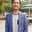Related Tags

d3

# What are axes in D3?Shahpar KhanD3 is an interactive JavaScript library for data visualization that uses Scalar Vector Graphics (SVG), HTML, and CSS to display charts and figures for illustrating numeric data. The most important part of displaying charts and figures is the axis. This shot will go through a step-by-step guide on how to draw an axis using D3.

### Step 1: Create SVG

First, you need to create a SVG with the appropriate width and height. To create an SVG element, make a var and then append the svg element with appropriate dimensions.

var svg = d3.select("body")
.append("svg")
.attr("width", 300)
.attr("height", 300);


### Step 2: Decide scale

D3 provides a wide selection of scale that includes scaleLinear, scaleLog, scalePoint, etc. Click here to see the different types of scales provided by D3. For this example, we will use scaleLinear.

We need to define the domainthe values that will be displayed on the axis - and the rangethe location where the axis will be placed.

var xscale = d3.scaleLinear()
.domain([1, 10])
.range([0, 100]);


## Step 3: Draw the axis

Finally, you need to set the position of the axis and specify the type. Below are the four types of axis:

Type Description
d3.axisTop() Creates horizontal axis at top
d3.axisBottom() Creates horizontal axis at bottom
d3.axisRight() Creates vertical axis at right
d3.axisLeft() Creates vertical axis at left

After doing so, append the axis to your svg variable and its done!

var xaxis = d3.axisBottom()
.scale(xscale);

svg.append("g")
.attr("transform", "translate(50,120)")
.call(xaxis);


## Combining the steps

The steps described above are combined below in an HTML code. A y-axis has been added to demonstrate how to make a complete 2-D axis. You can make the y-axis by following the steps above.

RELATED TAGS

d3

CONTRIBUTORShahpar Khan